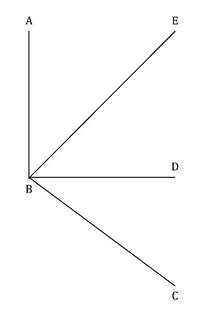Courses

#In the figure above, what is the value of angle ∠ABC?I. BD and BE are the angle bisectors of ∠CBE and ∠ABD respectively.II. ∠CBD = 45oa)Statement (1) ALONE is sufficient, but statement (2) alone is not sufficient to answer the question asked.b)Statement (2) ALONE is sufficient, but statement (1) alone is not sufficient to answer the question asked.c)BOTH statements (1) and (2) TOGETHER are sufficient to answer the question asked, but NEITHER statement ALONE is sufficient to answer the question asked.d)EACH statement ALONE is sufficient to answer the question asked.e)Statements (1) and (2) TOGETHER are NOT sufficient to answer the question asked, and additional data specific to the problem are needed.Correct answer is option 'C'. Can you explain this answer? Related Test: Test: Line And Angles

## GMAT Question

By Onokurhefe Festus · 3 days ago ·GMAT
Ankit Dixit answered Aug 30, 2019
Option c is correct

Don't assume ABD as 90deg as it's not mentioned.
both statements are not individually sufficient
combining we get value as 90+45=135